# Custom Source Time Functions

Copy
%matplotlib inline
%config Completer.use_jedi = False
# Standard Python packages
import pathlib

# Third-party imports.
import numpy as np
import matplotlib.pyplot as plt
import pyasdf

# Salvus packages
import salvus_flow.api
import salvus_flow.simple_config as sc
from salvus_mesh.simple_mesh import basic_mesh

## Main Message

Salvus offers a number of parameterized source time functions (STFs) - if that is not sufficient one can construct a custom one from a numpy array as illustrated in the following cell. Several rules apply:

• The array has to have the shape [npts, N] where npts the number of samples and N is the number of source components. The order can also be reversed in which case the array will automatically be transposed by SalvusFlow before the simulations are run.
• The spatial weights (f[_x, ...], m_[xx, yy, ...]) are always multiplied on top of the given STFs. Thus either normalize these or set the weights all to 1.0.
• For sources that have multiple components, e.g. vectorial or moment tensor sources, N is either equal to the number of independent source components (xy[z] in the vectorial case, m_xx, m_yy, ... in the tensor case) or equal to 1 in which case a copy of that array will be created before the weights are applied.
• The order of the given components must either be xy[z] in the vectorial case or adhering to the Voigt notation in the tensor case.
• Potentially specified rotation matrizes for the sources are applied after the weights and original STFs have been multiplied.
# A single array.
stf = sc.stf.Custom.from_array(
np.sin(np.linspace(0, 4 * np.pi, 100)),
sampling_rate_in_hertz=2.0,
start_time_in_seconds=0.0,
)
stf.plot()

# Combine with a source object to create a complete source object.
# Note the different weights will define the final source together
# with the STF.
src = sc.source.cartesian.VectorPoint2D(
x=10.0, y=0.0, fx=1e-2, fy=-2e-2, source_time_function=stf
)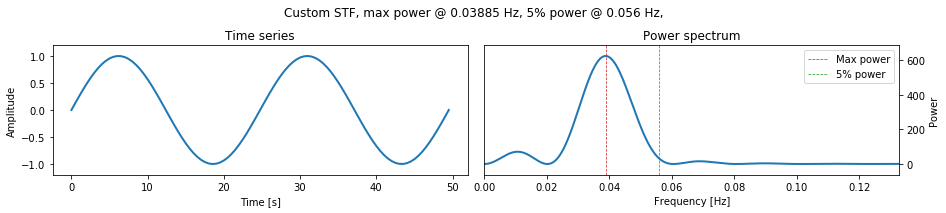# It is also possible to specify a separate array for every components.
array = np.array(
[
1.5 * np.sin(np.linspace(0, 4 * np.pi, 100)),
-3.0 * np.sin(np.linspace(0, 4 * np.pi, 100)),
2.3 * np.sin(np.linspace(0, 8 * np.pi, 100)),
]
)

stf = sc.stf.Custom.from_array(
array, sampling_rate_in_hertz=2.0, start_time_in_seconds=-10.0
)
stf.plot()

# Note that in this case the weights should be set to 1.0
src = sc.source.cartesian.VectorPoint2D(
x=10.0, y=0.0, fx=1.0, fy=1.0, source_time_function=stf
)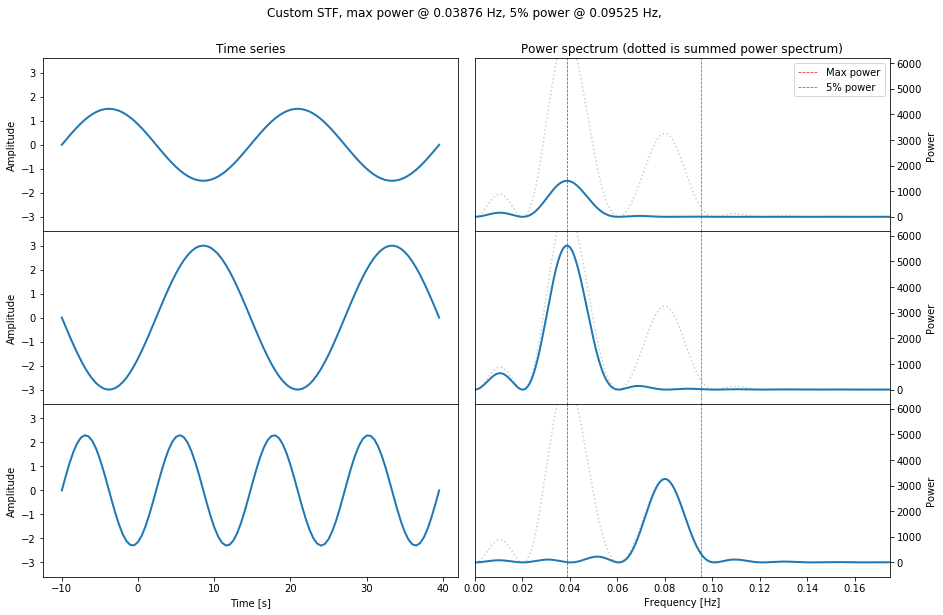## Expanded Tutorial

### Introduction

Salvus comes with a few wavelets that can readily be used as source time functions. However, sometimes it is necessary to define a custom source time function. This tutorial shows how to do that.

### Ricker wavelet as custom source time function

The Ricker wavelet is the second derivative of a Gaussian, and defined as

$s(t) = \left(1 - 2\,t^2\,\pi^2\,\omega^2\right) \, \exp\left(-t^2\,\pi^2\,\omega^2\right),$

where $t$ is the time and $\omega$ is the center frequency.

To see the connection with a Gaussian, it helps to define

$\sigma = \left(\pi\,\omega\right)^{-1},$

which gives

$s(t) = \left(1 - \frac{2\,t^2}{\sigma^2}\right) \, \exp\left(-\frac{t^2}{\sigma^2}\right).$

$s(t)$ is centered around zero, so we either have to introduce a time shift, or start the simulation at a time $t < 0$.

There are two important things to notice:

• To avoid artifacts in the wavefield the custom source function should always start smoothly from zero to be compatible with the homogeneous initial conditions.
• You don't need to worry too much about the correct sampling rate, as the source time function will be resampled internally using the actual time step of the simulation. Just make sure that you have sufficiently many data points to avoid undersampling.
wavelet_width_in_seconds = 0.1075
time_step_in_seconds = 1e-3
center_frequency = 14.5

sigma_2 = 1 / (np.pi * center_frequency) ** 2

time = np.linspace(
-wavelet_width_in_seconds,
wavelet_width_in_seconds,
int((2 * wavelet_width_in_seconds / time_step_in_seconds)),
)

sampling_rate_in_hertz = 1.0 / time_step_in_seconds

wavelet = (1 - (2 * time ** 2) / sigma_2) * np.exp(-time ** 2 / sigma_2)

# plot the wavelet
plt.plot(time, wavelet)
plt.xlabel("time [s]")
plt.ylabel("amplitude")
plt.title("Custom ricker wavelet")
plt.show()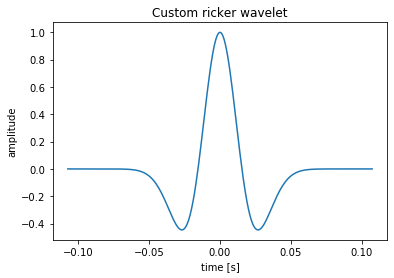### Simulation setup

To run a simulation with our custom source time functions, we'll just use a very simple example mesh.

# Simple 2D elastic mesh.
mesh = basic_mesh.CartesianHomogeneousIsotropicElastic2D(
vp=3200.0,
vs=1847.5,
rho=2200.0,
x_max=2000.0,
y_max=1000.0,
max_frequency=25.0,
).create_mesh()

receiver = sc.receiver.cartesian.Point2D(
x=1400.0, y=700.0, station_code="XX", fields=["displacement"]
)

Now we'll demonstrate the creation of the same source plus source time function in three different ways:

1. Using a built-in parameterized source time function.
2. Using a single component custom source time function and corresponding weights.
3. Using a multi-component source time function.
# Spatial weights of the vectorial source.
fx = 1e-5
fy = -0.8e-4

# Location.
sx = 1000.0
sy = 500.0

# Option 1 - Parameterized STF.
stf_1 = sc.stf.Ricker(center_frequency=14.5)
source_1 = custom_source = sc.source.cartesian.VectorPoint2D(
x=sx, y=sy, fx=fx, fy=fy, source_time_function=stf_1
)

# Option 2 - single-component STF and associated weights.
stf_2 = sc.stf.Custom.from_array(
array=wavelet,
sampling_rate_in_hertz=sampling_rate_in_hertz,
start_time_in_seconds=time,
)
source_2 = sc.source.cartesian.VectorPoint2D(
x=sx, y=sy, fx=fx, fy=fy, source_time_function=stf_2
)

# Option 3 - multi-component STF and unit weights.
source_time_function = [wavelet * fx, wavelet * fy]
stf_3 = sc.stf.Custom.from_array(
array=source_time_function,
sampling_rate_in_hertz=sampling_rate_in_hertz,
start_time_in_seconds=time,
)
source_3 = sc.source.cartesian.VectorPoint2D(
x=sx, y=sy, fx=1.0, fy=1.0, source_time_function=stf_3
)
stf_1.plot()
stf_2.plot()
stf_3.plot()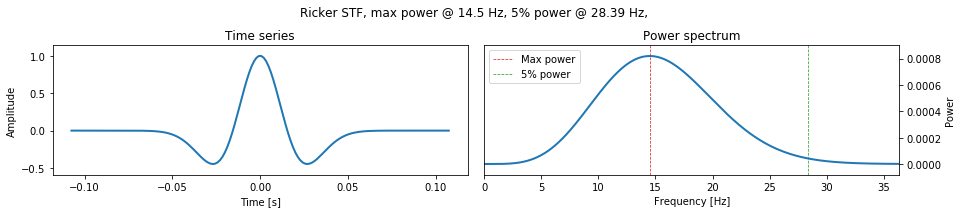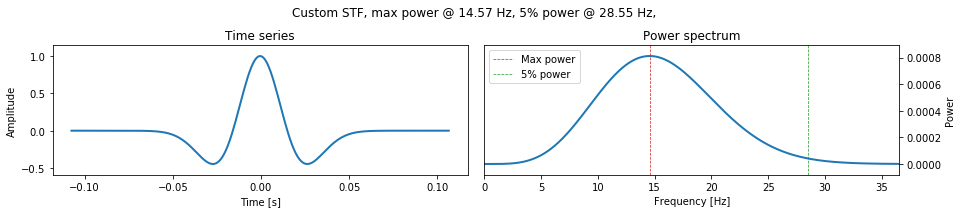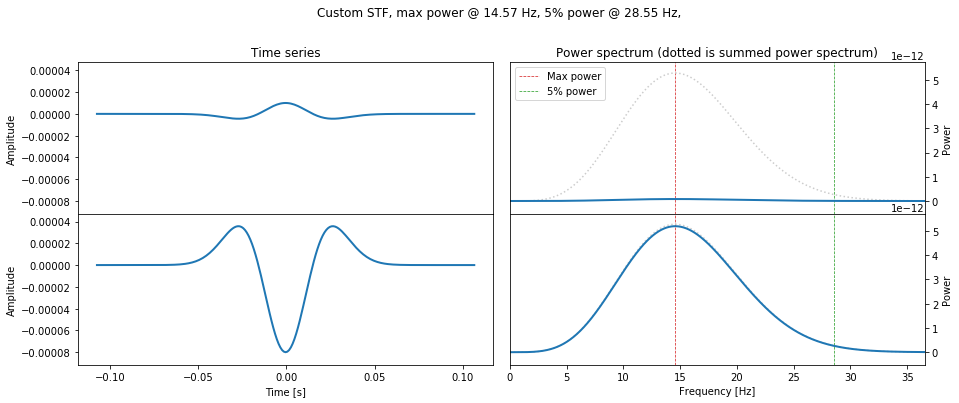Now it is time to actually run the simulations.

for _i, src in enumerate([source_1, source_2, source_3]):
sim.physics.wave_equation.end_time_in_seconds = 0.5

salvus_flow.api.run(
site_name="local",
input_file=sim,
ranks=1,
output_folder=f"output_custom_{_i}",
overwrite=True,
)
Job job_1910230020871670_6d64117185 running on local with 1 rank(s).
Site information:
* Salvus version: 0.10.6
* Floating point size: 32


* Downloaded 17.6 KB of results to output_custom_0.
* Total run time: 1.28 seconds.
* Pure simulation time: 0.99 seconds.
Job job_1910230020136522_a48b3e3743 running on local with 1 rank(s).
Site information:
* Salvus version: 0.10.6
* Floating point size: 32

* Downloaded 17.7 KB of results to output_custom_1.
* Total run time: 1.48 seconds.
* Pure simulation time: 1.23 seconds.
Job job_1910230020612493_75a704d85c running on local with 1 rank(s).
Site information:
* Salvus version: 0.10.6
* Floating point size: 32

* Downloaded 17.7 KB of results to output_custom_2.
* Total run time: 1.57 seconds.
* Pure simulation time: 1.31 seconds.


### Compare results

_, axes = plt.subplots(nrows=1, ncols=2, figsize=(15, 5))

for _i in range(3):
folder = pathlib.Path(f"output_custom_{_i}")
with pyasdf.ASDFDataSet(folder / "receivers.h5") as ds:
for _j, ax in enumerate(axes):
tr = ds.waveforms.XX_XX.displacement[_j]
ax.plot(tr.times(), tr.data, label=f"Source {_i}")
ax.set_title(f"Component {_j + 1}")
axes.legend()
axes.legend()
plt.show()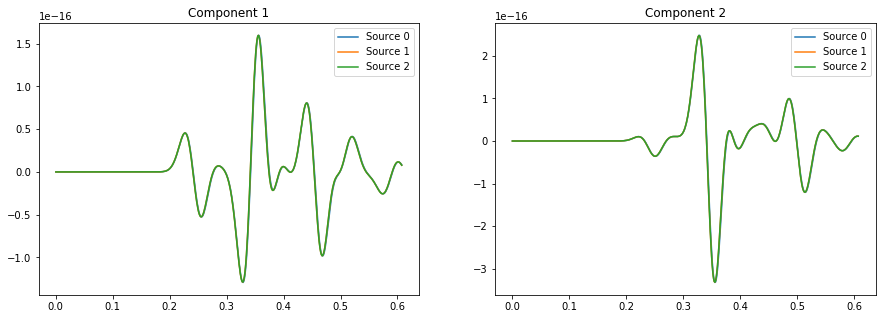As expected the traces look exactly the same. If you modify any of the parameters of any of the three sources you will see a difference.PAGE CONTENTS